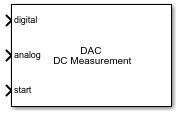# DAC DC Measurement

Measure DC performance metrics of DAC output

Since R2020a

•Libraries:
Mixed-Signal Blockset / DAC / Measurements & Testbenches

## Description

The DAC DC Measurement block measures DAC DC performance metrics such as offset error, gain error, integral nonlinearity (INL), and differential nonlinearity (DNL) errors. You can use the DAC DC Measurement block to validate the DAC architecture models provided in Mixed-Signal Blockset™, or you can use a DAC of your own implementation.

## Ports

### Input

expand all

Digital signal from a DAC, specified as a scalar.

Data Types: `fixed point` | `single` | `double` | `int8` | `int16` | `int32` | `uint8` | `uint16` | `uint32`

Converted analog signal from a DAC, specified as a scalar.

Data Types: `double`

External clock to start conversion, specified as a scalar. This port determines when digital-to-analog conversion process starts.

Data Types: `double`

## Parameters

expand all

Polarity of the input signal to the DAC.

#### Programmatic Use

 Block parameter: `Polarity` Type: character vector Values: `Bipolar`|`Unipolar` Default: `Bipolar`

Reference voltage of the DAC, specified as a real scalar in volts. Reference (V) helps determine the output from the input digital code, Number of bits, and Bias (V) using the equation:

.

#### Programmatic Use

 Block parameter: `Ref` Type: character vector Values: real scalar Default: `2`

Data Types: `double`

Bias voltage added to the output of the DAC, specified as a real scalar in volts. Bias (V) helps determine the output from the input digital code, Number of bits, and Reference (V) using the equation:

.

#### Programmatic Use

 Block parameter: `Bias` Type: character vector Values: real scalar Default: `0`

Data Types: `double`

The time required for the output of the DAC to settle to within some fraction of its final value, specified as a nonnegative real scalar in seconds.

#### Programmatic Use

 Block parameter: `SettlingTime` Type: character vector Values: real scalar Default: `3e-7`

Data Types: `double`

Delay before measurement analysis to avoid corruption by transients, specified as a nonnegative real scalar in seconds.

#### Programmatic Use

 Block parameter: `HoldOffTime` Type: character vector Values: nonnegative real scalar Default: `1e-3`

Data Types: `double`

Number of bits in the input word, specified as a unitless positive real integer. Number of bits determines the resolution of the DAC.

#### Programmatic Use

 Block parameter: `NBits` Type: character vector Values: positive real integer Default: `10`

Data Types: `double`

Frequency of the internal start conversion clock, specified as a real scalar in Hz. The Start conversion frequency parameter determines the conversion rate at the start of conversion.

#### Programmatic Use

 Block parameter: `StartFreq` Type: character vector Values: positive real scalar Default: `1e6`

Data Types: `double`

Minimum time the simulation must run to obtain meaningful results, specified as a positive real scalar in seconds.

To measure DC performance, the simulation must run so that the DAC can sample each digital code 20 times. Based on this assumption, the Recommended simulation stop time (s) T is given by:

,

where StartFreq is the frequency of the conversion start clock and Nbits is the resolution of the DAC.

The number of samples per bit is calculated using the equation:

.

This parameter is only reported by the block and is not editable.

Data Types: `double`

Measure the differential nonlinearity (DNL) error and integral nonlinearity (INL) error using the endpoint method. This method uses the endpoints of the actual transfer function to measure the DNL and INL errors.

Measure the differential nonlinearity (DNL) error and integral nonlinearity (INL) error using the best fit method. This method uses a standard curve-fitting technique to find the best fit to measure the DNL and INL errors.

Select to store detailed test results to a `struct` in the base workspace for further processing at the end of simulation. By default, this parameter is deselected.

Name of the variable that stores detailed test results, specified as a character string.

#### Dependencies

To enable this parameter, select Output result to base workspace parameter.

#### Programmatic Use

 Block parameter: `VariableName` Type: character vector Values: character string Default: `dac_dc_out`

Click to plot measurement result for further analysis.